A data structure is said to be linear if its elements form a sequence or a linear list. Previous linear data structures that we have studied like an array, stacks, queues and linked lists organize data in linear order. A data structure is said to be non linear if its elements form a hierarchical classification where, data items appear at various levels.

Trees and Graphs are widely used non-linear data structures. Tree and graph structures represents hierarchical relationship between individual data elements. Graphs are nothing but trees with certain restrictions removed.

we will explain special type of trees known as binary trees, which are easy to maintain in the computer.
TREES:
A tree is hierarchical collection of nodes. One of the nodes, known as the root, is at the top of the hierarchy. Each node can have at most one link coming into it. The node where the link originates is called the parent node. The root node has no parent. The links leaving a node (any number of links are allowed) point to child nodes. Trees are recursive structures. Each child node is itself the root of a sub-tree. At the bottom of the tree are leaf nodes, which have no children.

Trees represent a special case of more general structures known as graphs. In a graph, there is no restrictions on the number of links that can enter or leave a node, and cycles may be present in the graph. The figure below shows a tree and a non-tree.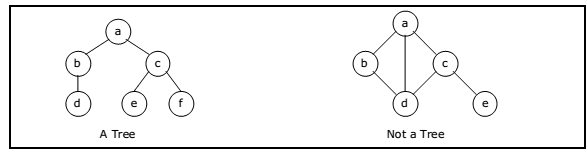In a tree data structure, there is no distinction between the various children of a node i.e., none is the “first child” or “last child”. A tree in which such distinctions are made is called an ordered tree, and data structures built on them are called ordered tree data structures. Ordered trees are by far the commonest form of tree data structure.

BINARY TREE:
In general, tree nodes can have any number of children. In a binary tree, each node can have at most two children. A binary tree is either empty or consists of a node called the root together with two binary trees called the left subtree and the right subtree.

A tree with no nodes is called as a null tree. A binary tree is shown in figure below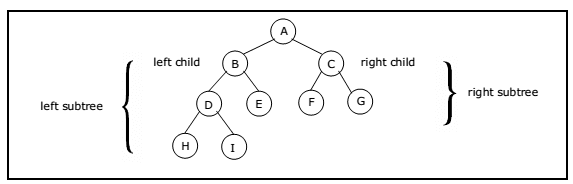Binary trees are easy to implement because they have a small, fixed number of child links. Because of this characteristic, binary trees are the most common types of trees and form the basis of many important data structures.

Tree Terminology:
Leaf node
A node with no children is called a leaf (or external node). A node which is not a leaf is called an internal node.

Path
A sequence of nodes n1, n2, . . ., nk, such that ni is the parent of ni + 1 for i = 1, 2,. . ., k – 1. The length of a path is 1 less than the number of nodes on the path. Thus there is a path of length zero from a node to itself. For the tree shown in figure 5.2.1, the path between A and I is A, B, D, I

Siblings
The children of the same parent are called siblings. For the tree shown in figure 5.2.1, F and G are the siblings of the parent node C and H and I are the siblings of the parent node D.

Ancestor and Descendant
If there is a path from node A to node B, then A is called an ancestor of B and B is called a descendant of A.

Subtree
Any node of a tree, with all of its descendants is a subtree.

Level
The level of the node refers to its distance from the root. The root of the tree has level O, and the level of any other node in the tree is one more than the level of its parent. For example, in the binary tree of Figure 5.2.1 node F is at level 2 and node H is at level 3. The maximum number of nodes at any level is 2n .

Height
The maximum level in a tree determines its height. The height of a node in a tree is the length of a longest path from the node to a leaf. The term depth is also used to denote height of the tree. The height of the tree of Figure 5.2.1 is 3.

Depth
The depth of a node is the number of nodes along the path from the root to that node. For instance, node ‘C’ in figure 5.2.1 has a depth of 1.

Assigning level numbers and Numbering of nodes for a binary tree:
The nodes of a binary tree can be numbered in a natural way, level by level, left to right. The nodes of a complete binary tree can be numbered so that the root is assigned the number 1, a left child is assigned twice the number assigned its parent, and a right child is assigned one more than twice the number assigned its parent. For example, see Figure 5.2.2.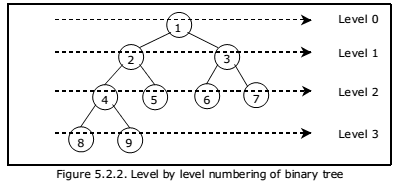Properties of binary trees:
Some of the important properties of a binary tree are as follows:

1. If h = height of a binary tree, then a. Maximum number of leaves = 2^h
2. Maximum number of nodes = 2^(h + 1) – 1
3. If a binary tree contains m nodes at level l, it contains at most 2m nodes at level l + 1.
4. Since a binary tree can contain at most one node at level 0 (the root), it can contain at most 2^l node at level l.
5. The total number of edges in a full binary tree with n node is n – 1.

Strictly Binary tree:
If every non-leaf node in a binary tree has nonempty left and right subtrees, the tree is termed as strictly binary tree. Thus the tree of figure 5.2.3(a) is strictly binary. A strictly binary tree with n leaves always contains 2n – 1 nodes.

Full Binary tree:
A full binary tree of height h has all its leaves at level h. Alternatively; All non leaf nodes of a full binary tree have two children, and the leaf nodes have no children.

A full binary tree with height h has 2^(h + 1) – 1 nodes. A full binary tree of height h is a strictly binary tree all of whose leaves are at level h. Figure 5.2.3(d) illustrates the full binary tree containing 15 nodes and of height 3. A full binary tree of height h contains 2^h leaves and, 2^h – 1 non-leaf nodes.

Thus by induction, total number of nodes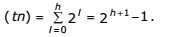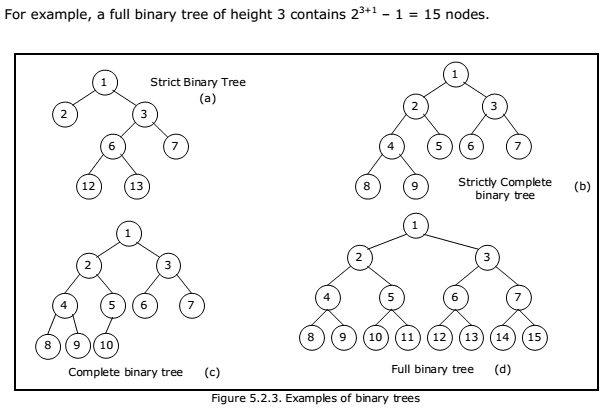Complete Binary tree:
A binary tree with n nodes is said to be complete if it contains all the first n nodes of the above numbering scheme. Figure 5.2.4 shows examples of complete and incomplete binary trees.

A complete binary tree of height h looks like a full binary tree down to level h-1, and the level h is filled from left to right.

A complete binary tree with n leaves that is not strictly binary has 2n nodes. For example, the tree of Figure 5.2.3(c) is a complete binary tree having 5 leaves and 10 nodes.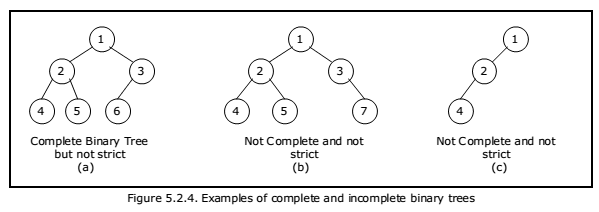Internal and external nodes:
We define two terms: Internal nodes and external nodes. An internal node is a tree node having at least one–key and possibly some children. It is some times convenient to have another types of nodes, called an external node, and pretend that all null child links point to such a node. An external node doesn’t exist, but serves as a conceptual place holder for nodes to be inserted.

We draw internal nodes using circles, with letters as labels. External nodes are denoted by squares. The square node version is sometimes called an extended binary tree. A binary tree with n internal nodes has n+1 external nodes. Figure 5.2.6 shows a sample tree illustrating both internal and external nodes.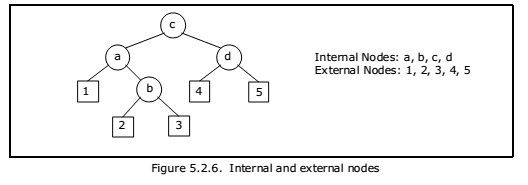Data Structures for Binary Trees:

1. Arrays; especially suited for complete and full binary trees.
2. Pointer-based.

Array-based Implementation:
Binary trees can also be stored in arrays, and if the tree is a complete binary tree, this method wastes no space. In this compact arrangement, if a node has an index i, its children are found at indices 2i+1 and 2i+2, while its parent (if any) is found at index floor((i-1)/2) (assuming the root of the tree stored in the array at an index zero).

This method benefits from more compact storage and better locality of reference, particularly during a preorder traversal. However, it requires contiguous memory, expensive to grow and wastes space proportional to 2h – n for a tree of height h with n nodes.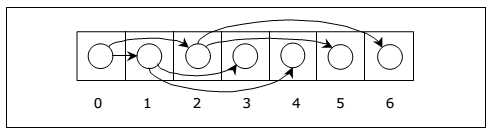Array representation is good for complete binary tree, but it is wasteful for many other binary trees. The representation suffers from insertion and deletion of node from the middle of the tree, as it requires the moment of potentially many nodes to reflect the change in level number of this node. To overcome this difficulty we represent the binary tree in linked representation. In linked representation each node in a binary has three fields, the left child field denoted as LeftChild, data field denoted as data and the right child field denoted as RightChild. If any sub-tree is empty then the corresponding pointer’s LeftChild and RightChild will store a NULL value. If the tree itself is empty the root pointer will store a NULL value.

• Insertion and deletion involve no data movement and no movement of nodes except the rearrangement of pointers.

• Given a node structure, it is difficult to determine its parent node.
• Memory spaces are wasted for storing NULL pointers for the nodes, which have no subtrees.

The structure definition, node representation empty binary tree is shown in figure 5.2.6 and the linked representation of binary tree using this node structure is given in figure 5.2.7.You may be interested in:
Data Structures and Algorithms – MCQs.
Data Structures and Algorithms Online Tests